Circuit Methodology

# Overview

## Abstract

The BioGear® Circuit Solver was created to extract the lumped parameter physics calculations from within the individual systems and centralize the generic calculations. Closed loop circuits can be defined easily within the Common Data Model (CDM). They are fully dynamic, and can be manipulated and modified at each time step. The solver can be used to analyze electrical, fluid, and thermal circuits using native units. The solver outputs were validated using the outputs from an existing known third party circuit solver. All results matched the validation data, confirming this is a sound approach for the BioGears Engine.

## Introduction

BioGears systems use lumped parameter circuits to mimic the physiology of the human body. These circuits use fluid or thermal elements that are analogous to electrical circuit elements. The circuits have several types of feedback mechanisms that can be set and changed at every time step. Figure 1 presents a generic example of very low fidelity lumped parameter physiology circuits. Circuits can be thought of as pipe networks for fluid analysis.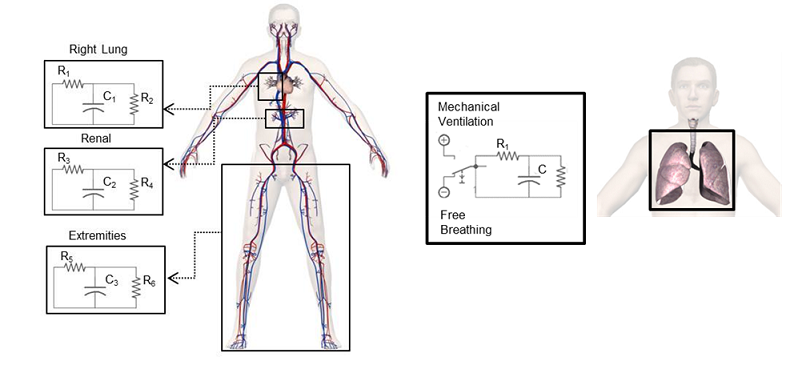Figure 1. An example of physiology lumped parameter modeling. This example shows very low fidelity models of specific cardiovascular compartments (left), and a respiratory combined mechanical ventilation and free breathing model (right) .

Note: For simplicity, this document uses electrical components and terminology when discussing the solver functionality. See Table 1 for analogies and details about the mapping of electrical components.

The BioGears CDM includes many of the same generic definitions traditionally used to define and analyze circuits. Paths are ideal conductor branches that may contain elements (i.e., resistors, capacitors, inductors, diodes, etc.). Nodes are junctions at the intersection of paths. Figure 2 shows these base circuit element definitions. Paths are each assigned one source and one target node. We use the convention of positive current from source to target when performing calculations.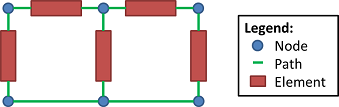Figure 2. Nodes and paths are the lowest level elements used to define all circuits in BioGears. Paths correspond to ideal conductors (i.e., wires). Nodes are placed at the intersections of paths. In fluid systems, paths can be thought of as frictionless pipes and nodes as pipe junctions.

# System Design

## Background and Scope

### Legacy Solution

The physiology engine from which BioGears was spawned (released by Advanced Simulation Corporation as Body Simulation for Anesthesia™) solved each lump parameter circuit using non-closed loop calculations contained within each system. The systems were extensively interconnected and the circuit calculations were unable to be decoupled. This made it extremely difficult to find sources of errors and inconsistencies in output results.

The circuit math was performed often using different approaches, with mixed results. It became apparent that neither energy nor mass was being truly conserved. This was true within the individual systems, as well as within the entire body. One-way fluid valves in the Cardiovascular System (similar to electrical diodes) would inadvertently allow backflow for a single time step when flows changed direction. Little validation was done to provide a sound fundamental base on which to build physiological systems.

### Requirements

Given the overall goals of BioGears, we set out to create a generic circuit solver on which to expand. The solver is required to efficiently solve transient linear and non-linear circuits. Some other high-level requirements include:

• Generic - All systems should be able to use the same basic circuit physics engine. This allows rapid development, and makes engine outputs much easier to validate and verify.
• Computational Speed - BioGears is required to maintain a transient full-body solution faster than real time on typical personal computers.
• Modular - Using the same basis for design and construction will aid in keeping the system decoupled.
• Extensible - We must take future growth into consideration and allow users and developers the proper tools and building blocks on which to add new functionality.
• Dynamic - Feedback mechanisms are required for each system. It is beneficial to be able to dynamically change, add, or remove circuits, sub-circuits, elements, and connections at run time.
• Conservation - We must uphold sound scientific principles and conserve energy, mass, and momentum.
• Common Data Model - The entire solution must reside within and effectively use the Common Data Model.
• Circuits types - BioGears will include fluid and thermal circuits. It is beneficial to use the same solver for both types of systems.
• Elements - The solver must be able to handle all base circuit elements (i.e., resistors, capacitors, inductors, etc.), with room for expansion.
• Open source - As a whole, any third-party software must adhere to the same license requirements as BioGears.

### Solutions Investigated

We considered several circuit solver options, including investigating existing software to determine if any would meet our needs. The best external code we found for integration into our C++ open source code was a variation on SPICE (Berkeley University’s Spice3f5), called Ngspice (http://ngspice.sourceforge.net/). Ngspice is an open source, general purpose circuit simulation program for nonlinear and linear analyses. It is developed with a C++ wrapper that maintains the original parametric netlist format (netlists can contain parameters and expressions) as inputs.

During implementation, we found Ngspice to be a good fit for our needs to solve closed-loop circuits, yet several negatives forced us to deviate from that path. We discovered a small number of bugs, some with possible workarounds. It was found to be very slow for our approach of solving a new (i.e., dynamic) circuit each time step. It was cumbersome to parse using the netlist input and output style. The most significant downside against implementing Ngspice into BioGears was the unavoidable build-up of memory that caused our software to crash.

Due to the aforementioned complications, we ultimately determined that creating our own generalized circuit analysis code was ideal and less labor-intensive than implementing existing software. This has the added benefit of allowing us to control every aspect of the analysis.

## Data Flow

Our generic Circuit Solver intentionally mirrors the same data flow found in each of the systems. Each time step consists of a Preprocess call to set up the circuits for analysis, a Process call to do the analysis, and a Post Process call to advance the time in preparation for the next time step.

### Preprocess

There is no literal Preprocess call within the circuit solver class (i.e., code). Each system individually and directly modifies its circuit(s). This allows our functionality to be entirely dynamic by performing a separate calculation every time step. See the Data Model Implementation Appendix below for details about BioGears circuit elements and the data they contain.

### Process

For the circuit-solving software to effectively calculate the nonlinear circuit states without human intervention, a robust, generic, and repeatable methodology is required. The solver performs numerical integration through linearization (first order approximations) by assuming a direct current (DC) solution for the given time step. The Modified Nodal Analysis (MNA) approach is used to determine the state of every node and path within the circuit. The steps for solving a circuit in a given time step are (also see Figure 3):

1. Perform numerical integration by using linearization (first order approximations) through MNA, using the matrix algebra equation:

$Ax = b$

Equation 1.

Where A is the matrix of constants, x is the vector of unknowns/variables, and b is the right side vector of knowns.

1. Use Kirkoff’s Current Laws (KCL) (sum of the currents is zero at each node) to populate the A matrix and b vector. Table 2 shows the equations used for determining flows, where flows (F) are equivalent to currents, and pressures (P) are equivalent to voltages.
2. Leverage the Eigen templated library (released by Tuxfamily) LU decomposition linear solver (FullPivLU) to solve for unknown variables (i.e., voltages and currents). This decomposition provides the generic approach to solving systems of linear equationsâ, and represents an LU decomposition of any matrix with complete pivoting. The matrix A is decomposed as:

$A=P^{-1} LUQ^{-1}$

Equation 2.

Where L is unit-lower-triangular, U is upper-triangular, and P and Q are permutation matrices. This is a rank-revealing LU decomposition. The eigenvalues (diagonal coefficients) of U are sorted in such a way that any zeros are at the end.

2. Calculate remaining unknown currents using the Trapezoidal Rule, where applicable. For nonlinear elements from time a to b, this is:

$\int _{a}^{b}f(x)dx\approx (b-a)\left[\frac{f(a)+f(b)}{2} \right]$

Equation 3.

3. Calculate diode currents using assumed open or closed switch states (cannot be solved directly). Iterate Steps 1–3 until a satisfactory solution is attained.
4. Calculate the change in charge (Q) across capacitors based on the capacitance (C) and voltage (V) change, and increment the total charge. The charge is conserved on the source and target nodes by incrementing/decrementing this amount. Selecting which node gains charge and which loses is done by the direction of the current in the path.

$Q(t)=C*V(t)$

Equation 4.

5. Invalidate the current on any path the user has specified to ignore. Note that this is to prevent unwanted transport to the reference node (i.e., ground).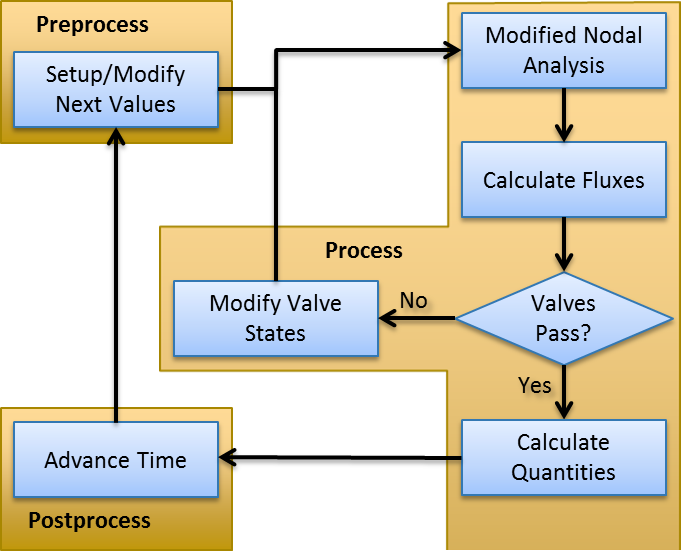Figure 3. Data flow chart showing the steps used at each time step to determine each circuit state.

There are several nuances for the handling of certain elements:

• Paths without elements are calculated as if they are zero voltage sources, which allows for direct solving for the current.
• Switches are modeled as element-less paths when closed and essentially infinite resistances (10100 ohms) when open.
• Valves (i.e., Diodes) are modeled as closed switches when the path source node voltage is higher than the target node (on) and as open switches when the voltage is greater on the target node (off).
• Polarized elements are modeled as an open switch when preventing polarity reversal

Because diode and polarized element states cannot be determined before solving a circuit, we are forced to make a considered guess. Therefore, at each time step, we begin with an assumed on/off state for each of these elements in the circuit. We begin with the previous time step solution to minimize the number of iterations. The entire circuit is solved using this assumed state, and the currents and voltages associated with the paths are considered. When a diode/polarized element is found to have an incorrect current direction or voltage reversed relative to its assumed state, the state is flipped. This process is then repeated until the valid solution is found. Intelligently choosing which diode states to switch is important because there are 2^(Number of Diodes/Polarized Elements) possible combinations in each circuit.

### Post Process

Post Process effectively advances time by moving the “next” time step parameter values to the “current” time step parameter values (see System Methodology).

## Features and Capabilities

### Approach and Extensibility

The BioGears systems use two different lumped parameter system types, each with analogies to electrical circuits. Most systems models (Cardiovascular, Respiratory, etc.) use fluid units (pressures, flows, and volumes), while others (Energy, Environment, etc.) use thermal units (temperature, heat transfer rate, and heat). Both model types use the same underlying physics to define relationships and solve for unknown values. We included the ability to calculate in native electrical units (voltage, current, and charge) to help with validation using known electrical circuit models and outputs. Table 1 lists system analogies and how they map to circuit elements.

Table 1. The various types of circuits that can be solved by the Circuit Solver. Some have units already defined and included in the CDM, while the others can be easily added. Possible inputs, outputs, elements, and parameters are all defined. Valves (diodes) and switches are not shown .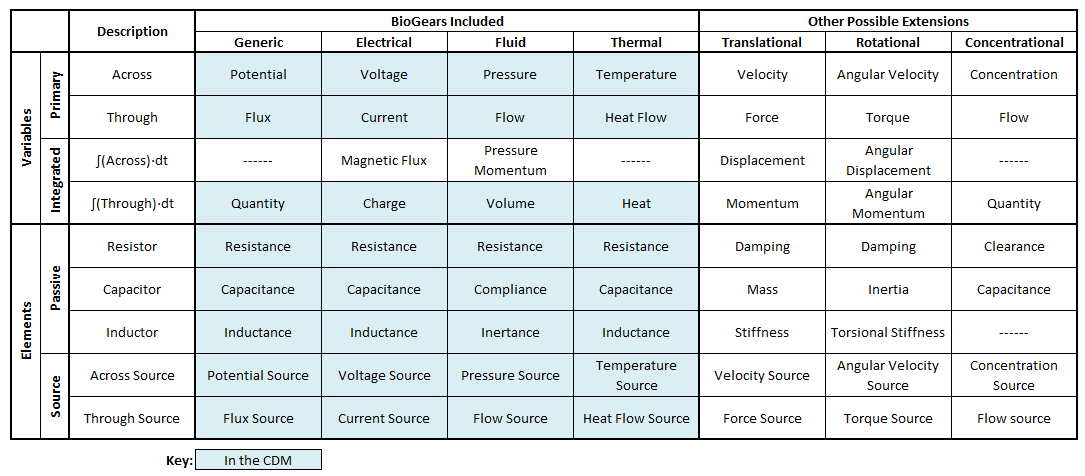While we designed the generic Circuit Solver to analyze our fluid, thermal, and electrical models, we can easily extend it to include any of the model types in Table 1. Further details specific to the implementation of our model with the hydraulic analogy are shown in Table 2. A more intuitive pipe analogy is described through images. The BioGears defined fluid model elements are outlined in the first column. The flow equations are important for our analysis technique outlined earlier.

Table 2. In-depth description of the hydraulic analogy for electrical circuits that are used extensively inside BioGears. The Elements are defined by the CDM and used by the solver. The Flow equations are important for solving for the unknown parameters.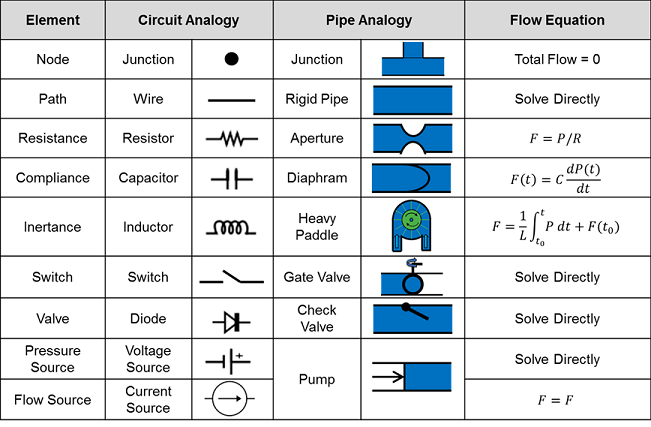The Circuit Solver is equipped to use all of the element types given in the second column of Table 2. All three passive element types (resistor, capacitor, and inductor) have a polarized element modeling option. When active, polarized elements will short the circuit when the target node voltage becomes greater than that of the source node. This allows the user to model electrolytic capacitors and further ensures fluid will not be added to hydraulic systems if compliances switch polarity.

### Data Model Implementation

Our mathematical approach to solving circuits is relatively straightforward, but our CDM implementation and integration with physiological models is novel. We implemented the Circuit Solver to use generic terms that are not specific to any one model type (see Table 1). Conversions to base units for each model are done in the background using the CDM unit conversion functionality. These base units are selected to prevent unnecessary conversion that would use critical computation resources, while still maintaining a direct mathematical relationship on both sides of the equation when performing the calculations.

The CDM allows us to implement the circuit math in a completely modular fashion. Every system uses the same physics, with the same CDM-defined elements. The burden on system developers is merely in setting up and manipulating the circuit correctly. A benefit to using a CDM is a significantly reduced development time.

The only significant requirement for circuit design is that it must be closed-loop. Beyond that, the solver can handle any combination of valid nodes, paths, and elements. Only one (or zero) element is allowed on each path and the convention of positive current going from source node to target node is used.

This sound foundation for defining and calculating circuit parameters, allows the engine to transport substances in a similarly generic fashion (see Drugs Methodology).

## Assumptions

### Circuits

There are several assumptions made for the circuit math in general:

• All circuits must be closed-loop
• All elements are idealized
• All circuits must have a reference node defined with a known voltage - unlike other circuit solvers, it does not necessarily have to have a potential value of zero
• Nonlinear sources need initial values. Therefore, paths with capacitors that do not have an initial voltage on the source or target nodes will assign it the reference node voltage value, and paths with inductors that do not have an initial current will be assigned a value of zero.

### Fluids

There are other assumptions specific to the BioGears fluid systems:

• Flows are incompressible
• Fluids are inviscid

## Limitations

There are frequency response limitations that are directly tied to the time step set externally to the solver itself. BioGears uses a time step of 1/50 s (~ 2 ms). Because the solver calculates nonlinear behavior through linearization using the trapezoid rule, extremely high frequency signal components will be lost. However, this far exceeds the Nyquist frequency for all current BioGears system models.

While it is not necessarily a limitation, users of the Circuit Solver must be careful to assign current and voltage source elements to set currents and voltages respectively. Attempting to directly set node voltages or path currents would result in an over-constrained solution. In these instances, the solver will overwrite those values.

# Results and Conclusions

## Validation

Beyond several numerical (hand) calculations used during implementation, we performed validation on the generalized circuit math by duplicating circuits in LTspice (version 4.21s, created by Linear Technology, Milpitas, CA). LTspice is based on the well-documented and validated SPICE simulator. We created circuits using all BioGears elements individually and in combination. We used several different types of dynamically-changing drivers to ensure proper transient functionality. The resulting voltage and current values were interpolated and validated to match for all 114 circuits. Table 3 shows a summary of the validation circuits investigated.

Table 3. The list of circuits created in BioGears and validated against LTspice. Every element is covered in combination with each other.
Test Name Purpose of test Results Summary
BadDiodeDC Intentionally failing to test Diode implementation LTSpice: Will not run, BioGears: successfully fails - provides feedback on failure
BadDiodePULSE Intentionally failing to test Diode implementation LTSpice: Will not run, BioGears: successfully fails - provides feedback on failure
BadDiodeSIN Intentionally failing to test Diode implementation LTSpice: Will not run, BioGears: successfully fails - provides feedback on failure
BasicCircuit Simple pressure source with resistances in series Match
BasicDiodeDCCurrent Diode behavior in response to a current source Match
BasicDiodePULSECurrent Diode behavior in response to a current source Match
BasicDiodeSINCurrent Diode behavior in response to a current source Match
Comprehensive1DC Combination of element types with a voltage source and a current source Match
Comprehensive1PULSE Combination of element types with a voltage source and a current source Match
Comprehensive1SIN Combination of element types with a voltage source and a current source Match
CurrentCompDC Combination of resistors and diode in response to a current source Match
CurrentCompPulse Combination of resistors and diode in response to a current source Match
CurrentCompSIN Combination of resistors and diode in response to a current source Match
ParallelCapDC Adding capacitors in parallel with a voltage source Match
ParallelCapDCCurrent Adding capacitors in parallel with a current source Match
ParallelCapPulse Adding capacitors in parallel with a voltage source Match
ParallelCapPULSECurrent Adding capacitors in parallel with a current source Match
ParallelCapSIN Adding capacitors in parallel with a voltage source Match
ParallelCapSINCurrent Adding capacitors in parallel with a current source Match
ParallelIndDC Adding inductors in parallel with a voltage source Match
ParallelIndDCCurrent Adding inductors in parallel with a current source Match
ParallelIndPULSE Adding inductors in parallel with a voltage source Match
ParallelIndPULSECurrent Adding inductors in parallel with a current source Match
ParallelIndSIN Adding inductors in parallel with a voltage source Match
ParallelIndSINCurrent Adding inductors in parallel with a current source Match
ParallelPressureSourceAdditionDC Intentionally failing adding voltage sources in parallel BioGears: successfully fails - provides feedback on failure
ParallelPressureSourceAdditionPULSE Intentionally failing adding voltage sources in parallel BioGears: successfully fails - provides feedback on failure
ParallelPressureSourceAdditionSIN Intentionally failing adding voltage sources in parallel BioGears: successfully fails - provides feedback on failure
ParallelRCDC Parallel resistor and capacitor response to a voltage source Match
ParallelRCPULSE Parallel resistor and capacitor response to a voltage source Match
ParallelRCSIN Parallel resistor and capacitor response to a voltage source Match
ParallelRDC Parallel resistor response to a voltage source Match
ParallelRDCCurrent Parallel resistor response to a current source Match
ParallelRLCDC Parallel resistor, inductor, and capacitor response to a voltage source Match
ParallelRLCDCCurrent Parallel resistor, inductor and capacitor response to a current source Match
ParallelRLCPULSE Parallel resistor, inductor, and capacitor response to a voltage source Match
ParallelRLCPULSECurrent Parallel resistor, inductor and capacitor response to a current source Match
ParallelRLCSIN Parallel resistor, inductor, and capacitor response to a voltage source Match
ParallelRLCSINCurrent Parallel resistor, inductor and capacitor response to a current source Match
ParallelRLDC Parallel resistor and inductor response to a voltage source Match
ParallelRLDCCurrent Parallel resistor and inductor response to a current source Match
ParallelRLPULSE Parallel resistor and inductor response to a voltage source Match
ParallelRLPULSECurrent Parallel resistor and inductor response to a current source Match
ParallelRLSIN Parallel resistor and inductor response to a voltage source Match
ParallelRLSINCentered Parallel resistor and inductor response to a voltage source Match
ParallelRLSINCurrent Parallel resistor and inductor response to a current source Match
ParallelRPULSE Parallel resistor response to a voltage source Match
ParallelRPulseCurrent Parallel resistor response to a current source Match
ParallelRSIN Parallel resistor response to a voltage source Match
ParallelRSINCurrent Parallel resistor response to a current source Match
SeriesCapDC Adding capacitors in series with a voltage source Match
SeriesCapDCCurrent Adding capacitors in series with a current source Match
SeriesCapPulse Adding capacitors in series with a voltage source Match
SeriesCapPULSECurrent Adding capacitors in series with a current source Match
SeriesCapSIN Adding capacitors in series with a voltage source Match
SeriesCapSINCurrent Adding capacitors in series with a current source Match
SeriesCurrentSourceAdditionDC Intentionally failing adding current sources in series BioGears: successfully fails - provides feedback on failure
SeriesCurrentSourceAdditionPULSE Intentionally failing adding current sources in series BioGears: successfully fails - provides feedback on failure
SeriesCurrentSourceAdditionSIN Intentionally failing adding current sources in series BioGears: successfully fails - provides feedback on failure
SeriesIndDC Adding inductors in series with a voltage source Match
SeriesIndDCCurrent Adding inductors in series with a current source Match
SeriesIndPULSE Adding inductors in series with a voltage source Match
SeriesIndPULSECurrent Adding inductors in series with a current source Match
SeriesIndSIN Adding inductors in series with a voltage source Match
SeriesIndSINCurrent Adding inductors in series with a current source Match
SeriesRCDC Series resistor and capacitor response to a voltage source Match
SeriesRCPULSE Series resistor and capacitor response to a voltage source Match
SeriesRCSIN Series resistor and capacitor response to a voltage source Match
SeriesRDC Series resistors response to a voltage source Match
SeriesRLCDC Series resistor, inductor and capacitor response to a voltage source Match
SeriesRLCDCCurrent Series resistor, inductor and capacitor response to a current source Match
SeriesRLCPULSE Series resistor, inductor and capacitor response to a voltage source Match
SeriesRLCPULSECurrent Series resistor, inductor and capacitor response to a current source Match
SeriesRLCSIN Series resistor, inductor and capacitor response to a voltage source Match
SeriesRLCSINCurrent Series resistor, inductor and capacitor response to a current source Match
SeriesRLDC Series resistor and inductor response to a voltage source Match
SeriesRLDCCurrent Series resistor and inductor response to a current source Match
SeriesRLPULSE Series resistor and inductor response to a voltage source Match
SeriesRLPULSECurrent Series resistor and inductor response to a current source Match
SeriesRLSIN Series resistor and inductor response to a voltage source Match
SeriesRLSINCurrent Series resistor and inductor response to a current source Match
SeriesRPULSE Series resistors response to a voltage source Match
SeriesRSIN Series resistors response to a voltage source Match
SimpleDiodeDC Diode behavior in response to a voltage source Match
SimpleDiodePULSE Diode behavior in response to a voltage source Match
SimpleDiodeSIN Diode behavior in response to a voltage source Match
SwitchRCDC Circuit behavior when a switch is thrown with a voltage source Match
SwitchRCDCCurrent Circuit behavior when a switch is thrown with a current source Match
SwitchRCPULSE Circuit behavior when a switch is thrown with a voltage source Match
SwitchRCPULSECurrent Circuit behavior when a switch is thrown with a current source Match
SwitchRCSIN Circuit behavior when a switch is thrown with a voltage source Match
SwitchRCSINCurrent Circuit behavior when a switch is thrown with a current source Match

The most interesting and complex circuits use a combination of all elements. Two of these comprehensive circuits are shown below because of their complexity. The first uses sine wave sources and the second uses pulse sources. There is a strong correlation between the given LTspice outputs and the calculated BioGears outputs. Note that the sign convention of the current across voltage sources is reversed for LTspice, because it does not maintain the source-to-target positive current standard as is done with BioGears.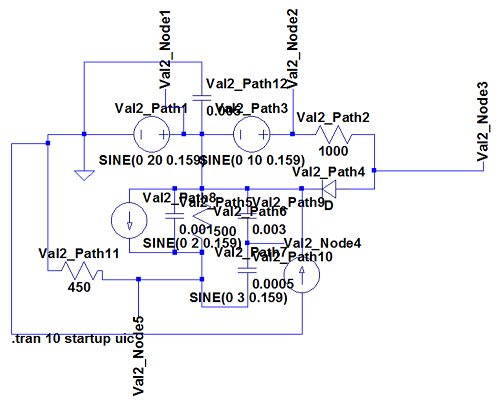Figure 4. The LTspice circuit diagram for the first comprehensive circuit that exemplifies the validation completed on the solver. Many different types of elements and multiple voltage and current sources are included. The same circuit was defined using the CDM for comparison.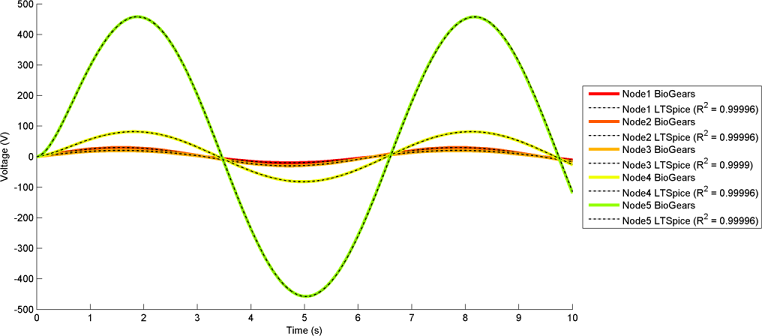Figure 5. BioGears node voltage outputs for the first comprehensive circuit compared to LTspice baseline values, using sinusoid sources. All are very tightly correlated.Figure 6. BioGears path current outputs for the first comprehensive circuit compared to LTspice baseline values. All are very tightly correlated. The signs of the current through voltage sources are reversed because of differing conventions for those elements.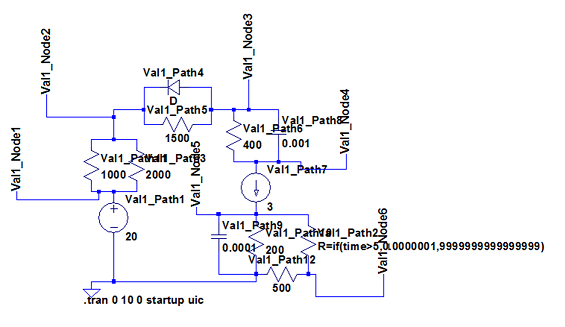Figure 7. The LTspice circuit diagram for the second comprehensive circuit that exemplifies the validation completed on the solver. Many different types of elements and multiple voltage and current sources are included. The same circuit was defined using the CDM for comparison.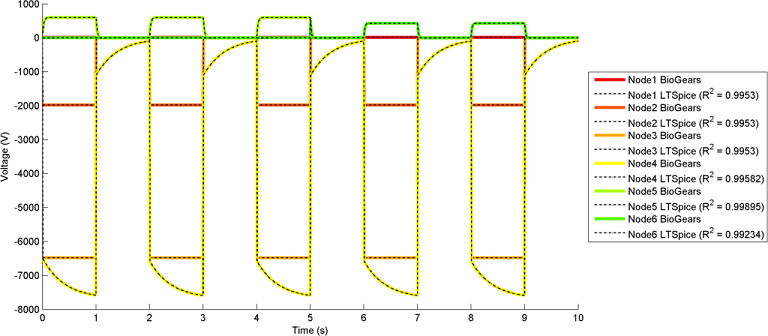Figure 8. BioGears node voltage outputs for the second comprehensive circuit compared to LTspice baseline values, using pulse sources. All are very tightly correlated.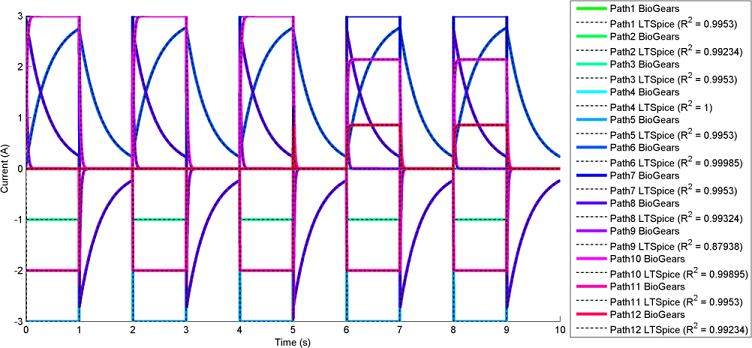Figure 9. BioGears path current outputs for the second comprehensive circuit compared to LTspice baseline values. All are very tightly correlated. The sign of the current through voltage sources are reversed because of differing conventions for those elements.

BioGears has been shown to successfully conserve mass, energy, and momentum within all defined closed-loop systems. The successful conservation of mass provided by the solver is shown in Figure 10. The volume (quantity/charge) within cardiovascular circuit nodes through approximately 2.5 full heart beat cycles. The total volume of all compartments remains at a constant value of 5L throughout the entire process.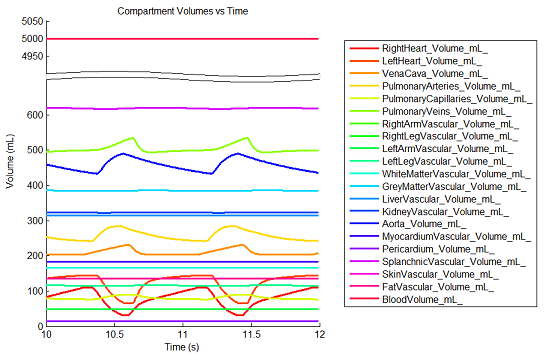Figure 10. The blood volume within each compartment continuously varies in the cardiovascular circuit. The sum of these volumes does not change at any time step, and system mass is successfully conserved.

All basic Circuit Solver functionality is further validated and verified with specific unit tests that target individual methods. The following functionality has been successfully validated by individual tests:

• Incorrect usage errors
• Combined circuits
• Dynamically-changing elements
• Dynamically-changing circuits
• Fluid, thermal, and electrical model types
• Non-zero reference node voltage
• Polarized elements
• Dynamically modifying capacitor charge

## Conclusion

The BioGears generalized circuit analysis techniques have successfully accomplished all of the goals for that area of the code. The solver implements the CDM effectively and generically in a way that allows for infinitely complex lumped parameter models that can also be combined. We made it simple to modify existing functionality and add new models. This has saved, and will continue to save, significant amounts of development time. The generic and modular design also allows for much easier bug finding and fixing, system parameter tuning, and system validation. The burden for the modeler is on creating a good model and not worrying about implementing sound physics math.

The Circuit Solver has also been designed such that it can be extracted from BioGears and used for any software that needs to perform transient circuit analysis.

# Future Work

## Coming Soon

The list below includes some of the planned functionality additions.

• Functionality to create port connections between circuits for improved modularity between systems/subsystems

## Recommended Improvements

Other functionality that may be beneficial includes:

• A sensitivity analysis and error term determination
• An analysis of frequency constraints
• More circuit elements (i.e., amplifiers, transformers, transistors, non-ideal elements, zener diodes, etc.)

# Appendices

## Data Model Implementation

SECircuit

SECircuitCalculator

SECircuitNode

SECircuitPath

## Acronyms

CDM - Common Data Model

DC - Direct Current

GUI - Graphical User Interface

KCL - Kirkoff's Current Law

MNA - Modified Nodal Analysis

SPICE - Simulation Program with Integrated Circuit Emphasis